# Multi-State Simulations, Part II

Click here to go to the previous lesson on multi-state file simulations

Suppose you wanted to do multi-state analysis on a set of measured S-parameter files that someone gave you? For example, measured S-parameters of the "primary" states of a five-bit attenuator would comprise a set of six files, the reference state, the 11.5, 22.5, 45, 90 and 180 states. You want to calculate the average loss of the states, and the RMS phase error, etc. The information on this page will help you accomplish this.

This came from Don a while ago, for which he was awarded the coveted Microwaves101 pocketknife. Turns out there is an "if statement" in ADS. We tried it, it works, even if it is Keysight's best kept secret...

Here is the syntax, which you can compare to what we describe below

B0= if Xstate=0 then 1 else 0 endif

B1=if Xstate=1 then 1 else 0 endif

Etc...

Thanks a lot for obsoleting all the sweet analysis on this page, Don! But we'll leave it up for posterity...

New for May 2017! Scott contributes another method in Keysight's ADS for "if statements":

B0=(Xstate==0) ? 1 : 0

Both allow for nested if statements:

B0=(Xstate==0) ? 1 : (Xstate==2) ? 1: 0

That’s not exactly a useful statement, but one case this is useable would be changing a variable over frequency, say third order intercept point:

Amp_nonlin = (freq < 50e6) ? 20 : (freq < 500e6) ? 30 : (freq < 5e9) ? 25: 10

TOI is 20 for frequencies less than 50MHz, 30 between 50-500MHz, 25 between 500MHz-5GHz, and 10 above 5GHz

This works in schematics and data display.

Getting into advanced territory, you could substitute the TOI value with a DataAccessComponent (DAC) column variable, requiring changing the real/integer values with strings.  Allows the use of multiple DACs to cover a range of attributes.

Thanks, Scott!

Previously we looked at using a parameter sweep to throw a binary sequence of bits, in a phase shifter design were all of the bits are accessible as individual two-port designs. In this next case, we are interested in turning on an arbitrary set of circuit files, one at a time, from a parameter sweep.

Yes, there is something called a multi-dimensional file that could be used, but if you don't understand it, it is another way to get in trouble, and it is somewhat painful to convert a set of individual files into an "mdf" file. Trust us, our way out of this mess is way more fun!

Let's let the parameter we are sweeping be "Xstate", which will control the simulation and generate S-parameters of a two-port circuit that are different for each Xstate value.

The sweep function is Xstate=0,1,2,3,4,5, ..., it is controlled by in integer start, stop and step size (in this case start=0, stop=5 and step=1)

Note that Xstate could just as well start at one, but "0" can be our reference state in a phase shifter or attenuator or whatever is being switched.

The state variables we generate are B1, B2, B3, B4, B5, B6...

Xstate B0 B1 B2 B3 B4 B5
0 1 0 0 0 0 0
1 0 1 0 0 0 0
2 0 0 1 0 0 0
3 0 0 0 1 0 0
4 0 0 0 0 1 0
5 0 0 0 0 0 1

etc.

If we had an "IF" statement, this would be sooooo easy.

IF(N=1, B1=1, else B1=0)

IF(N=2, B2=1, else B2=0)

and so on.

But in EDA software where you don't have an IF statement, you need a clever way to do this. We do have ABS (absolute value) and INT (integer) functions.

We'll show you one solution below. Perhaps you can come up with a simpler way, if you do we'll send you a free gift! Offer is now over thanks to Don....

## Example: Hittite HMC543 X-band phase shifter

You can download a zip file of the sixteen phase states of this four-bit phase shifter. We did!

Here is the function that creates the the "B values" that we will use to turn on the individual S2P files in a single two-port network. This is where the magic happens! If you want to play with this math, try it in Excel, it is much easier to manipulate.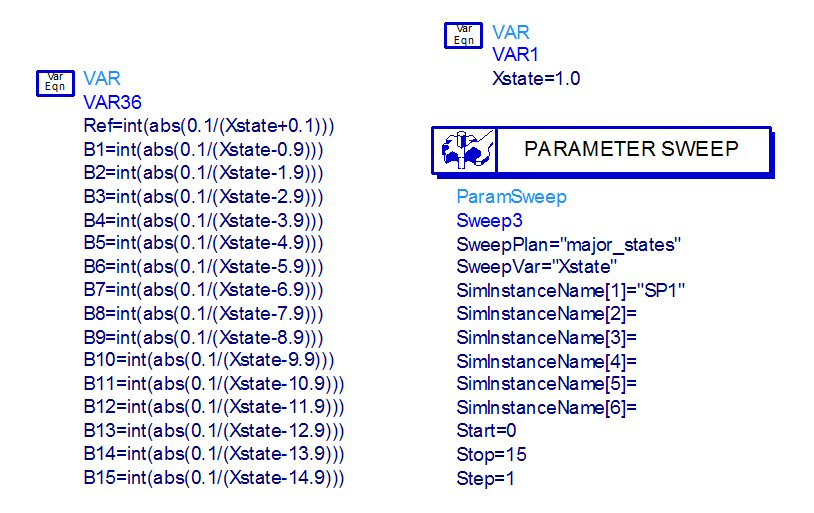These three 2-ports are needed to do some fancy-pants calculations for RMS errors and other parameters. S21 is the 2-port that will sweep through the phase states, S43 is the reference state (see "Ref=1" and all other B values are zero), and S65 is the expected phase value. The various states of the phase shifter are parameters that are fed into the circuit, with names "Ref", "State22", "State45", etc. Can you guess why we named them this way? If not, the Post Office is always hiring letter carriers... oops, maybe they aren't this year...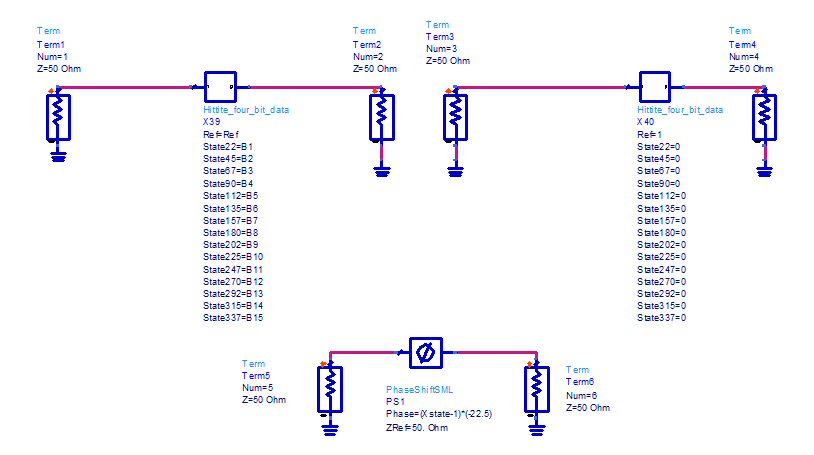If you could click into the circuit "Hittite_four_bit_data" you would see the following network. All sixteen sets of S-parameters are "called" by turning on the proper resistors (0 ohms) while turning off the other resistors (very high value ohms).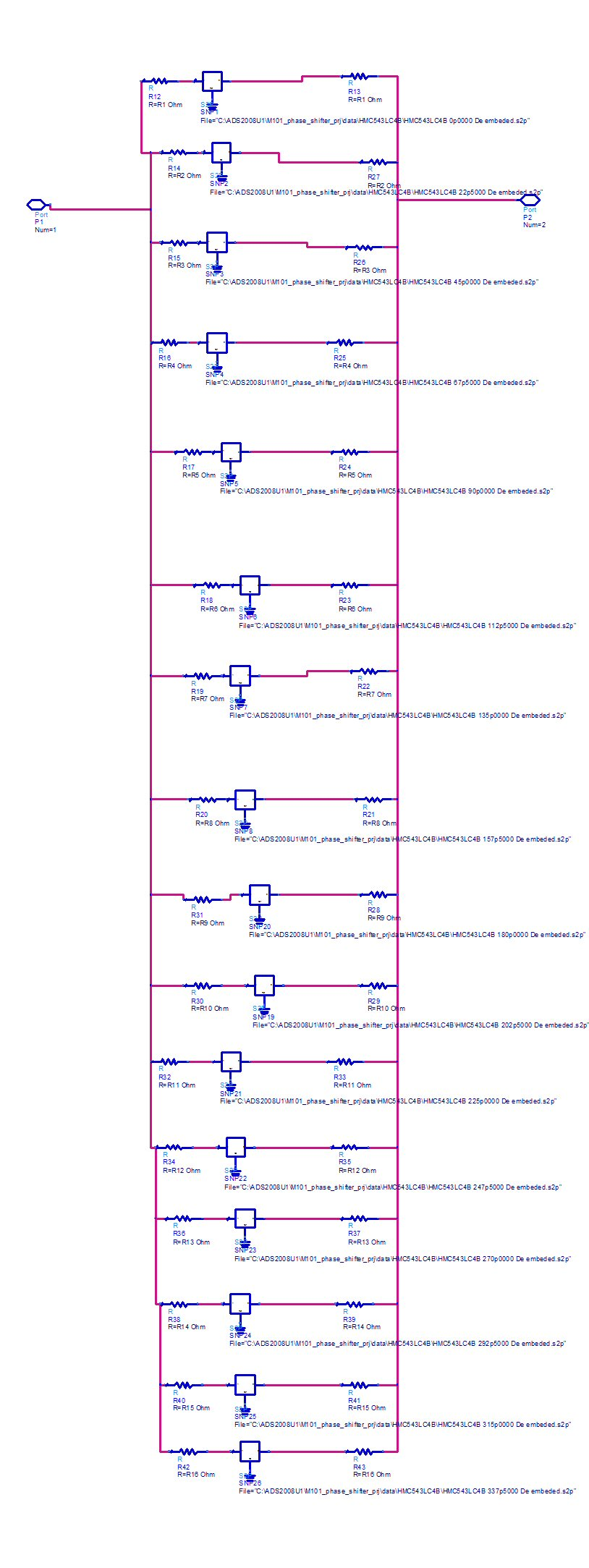The variable block below is what calculates the resistors from the B-values. Yes, we could have done this all in one step, but we'd rather deal with the "binaryness" of the B-values at the top level, rather than the 0/infinity values of resistors.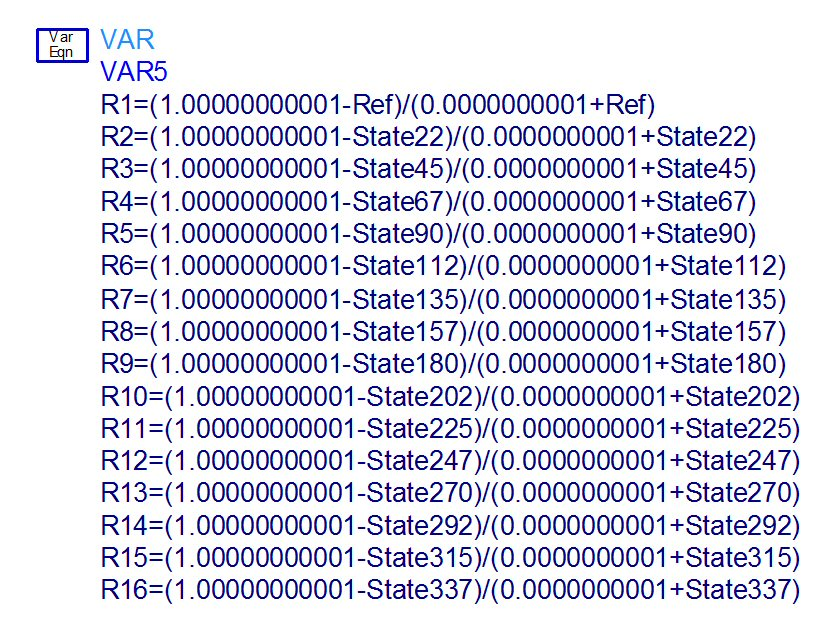Now let's run the analysis and look at the multistate data. The HMC543 phase shifter is advertised to work from 8 top 12 GHz but Hittite provides data down to 6 GHz. Below are the transmission coefficients of the 16 states. The amplitudes are spread to about a 1 dB window across much of the band, this is going to drive up the RMS amplitude error as we'll see below.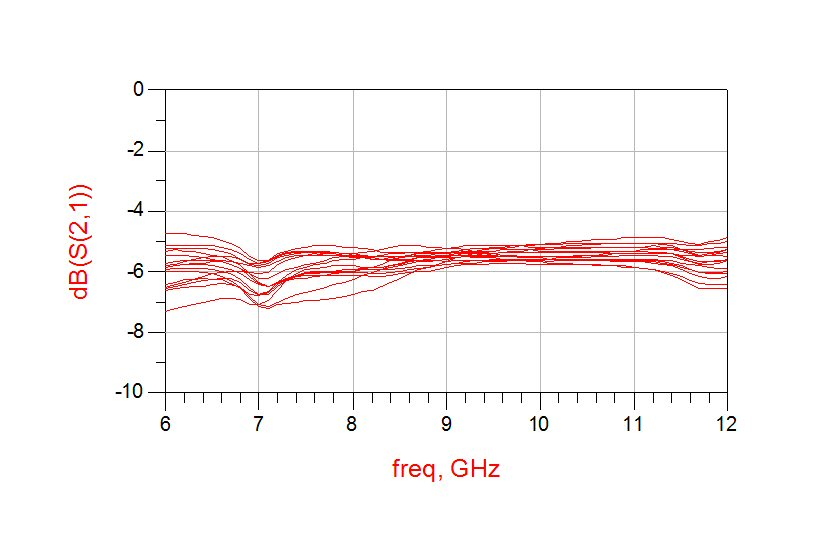Below are the return losses.(reflection coefficients in dB). You can see that the "kink" in insertion loss down at 7 GHz (above) is caused by mismatch (below).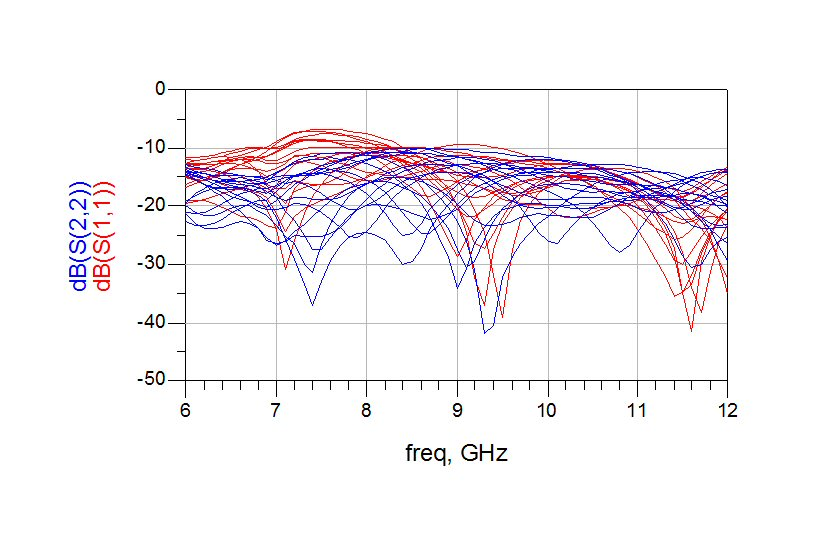Below are the raw phase states. From 8 GHz and upward they are monotonic (don't accidentally cross), a sign of a good phase shifter design. Give that designer a raise!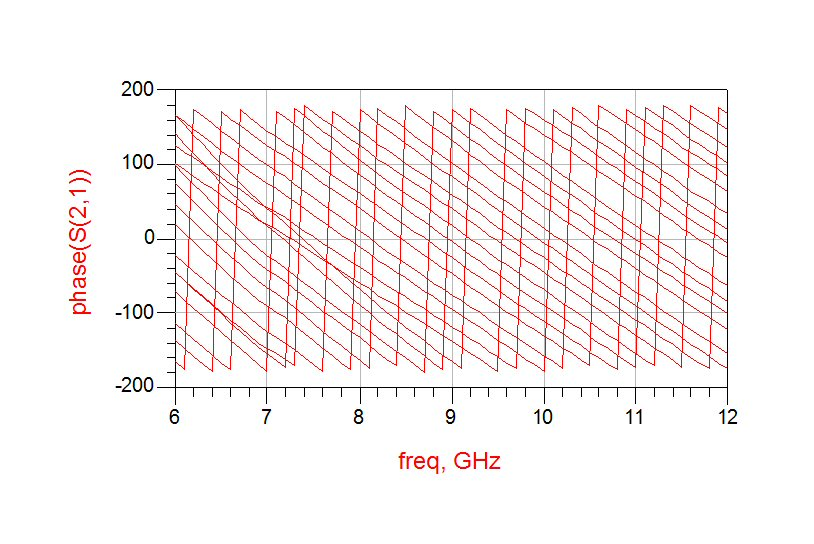Below we've unwound the phase and subtracted the shifted states from the reference state. What is wrong with this picture? It should be plotted with the Y-axis in increments of 45 or 90 degrees so you can quickly see how the states are lining up to their intended values. The phase states are very flat from 8 GHz and upward, a characteristic of high-pass/low-pass phase bit structures, and satisfies a very important phase shifter characteristic for phased arrays.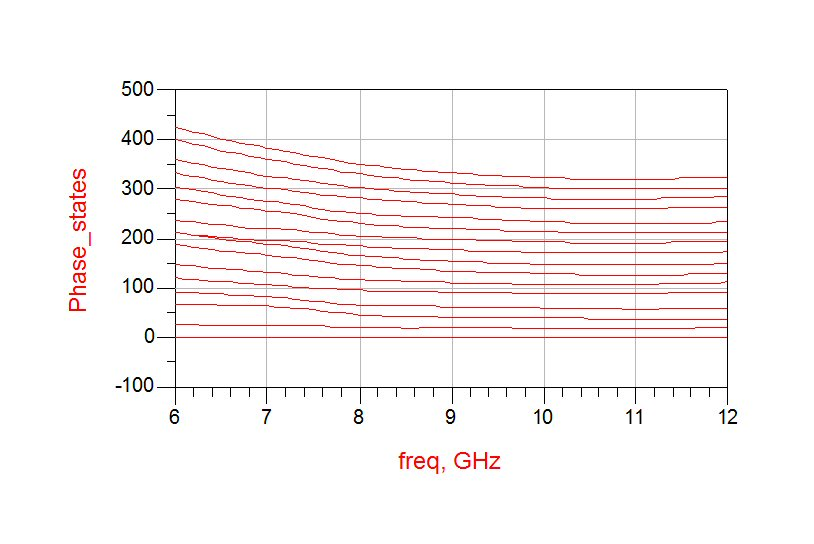We're going to skip some intermediate steps and plot the RMS errors. There are two ways to RMS the phase error. The fool's way is to subtract each state from the reference state (red) and RMS the fifteen shifted states. The better way is to calculate an "ideal reference state" (actually, the mean of all the states) and RMS all sixteen states (blue). If you had Hittite's data sheet in front of you you'd see that they do it the correct way. This phase shifter provides less than 5 degrees RMS error from 8 to 12 GHz. Sweet!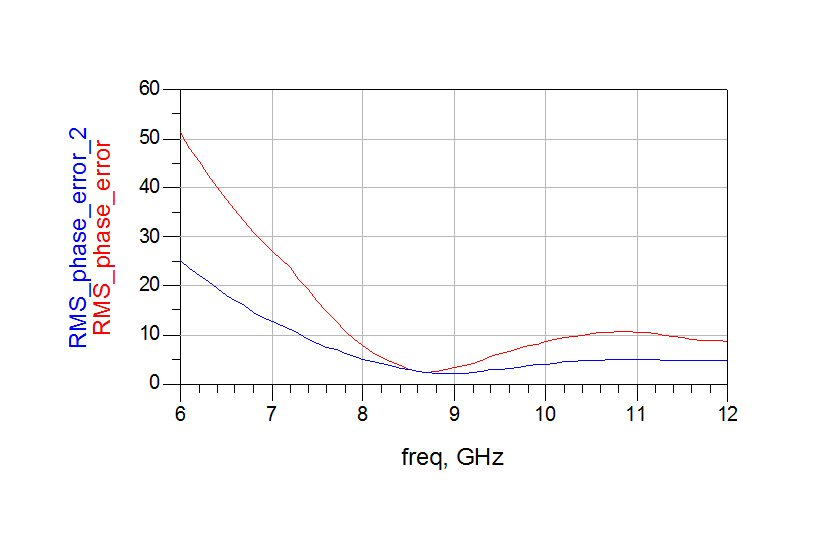Below we have plotted the RMS amplitude error (which does not appear on the data sheet). Pretty good, but it is possible to do better. Send us a check with at least three zeros to the left of the decimal point and we'll sit in on the redesign review!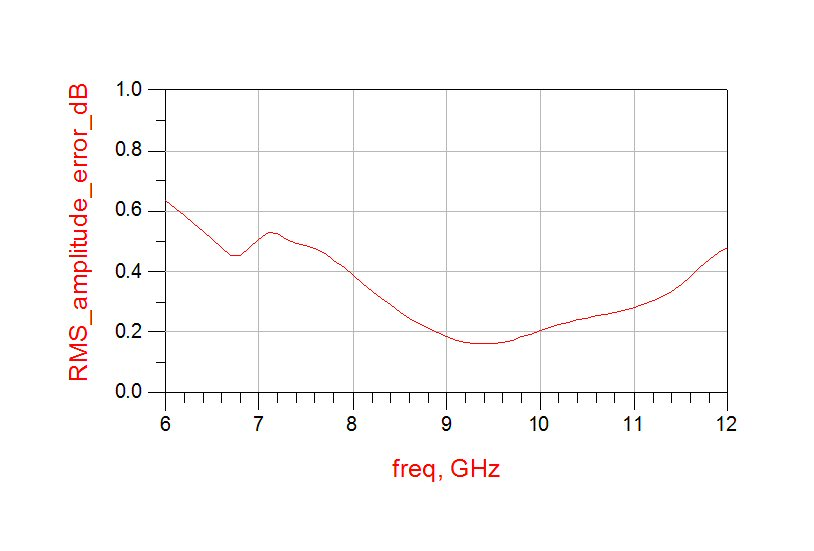Overall we give this circuit a B+ letter grade, just marked it down a little for the amplitude spreading...

Author : Unknown Editor